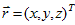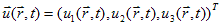# Theory of physical vacuum (ether)

Postulate. All fields and material objects in the Universe are various perturbations of physical vacuum, which is dense compressible inviscid medium in three-dimensional Euclidean space with coordinates, having in every time station t densityand perturbation propagation velocity vector.

Therefore, in compliance with Newtonian mechanics equations of physical vacuum dynamics should be as followswhere first equation is an equation of continuity, and second is the momentum equation.

It is shown, that all basic equations of classical electrodynamics, theory of elementary particles and gravitation theory can be derived from these two nonlinear equations of dynamics of physical vacuum. Thereby, it is shown that a set of generally unrelated geometric, algebraic and stochastic linear theories of modern physics, which are fudged to agree with experimental data and operating with concepts of multidimensional spaces and space-time continuums, can be replaced with one nonlinear theory of physical vacuum in ordinary three-dimensional Euclidean space, based exclusively on laws of classical mechanics which are invariant concerning Galilean transformations.

References to the three presently developed parts of the theory of physical vacuum (ether) are presented below: